Ex 3.1

Chapter 3 Class 10 Pair of Linear Equations in Two Variables
Serial order wise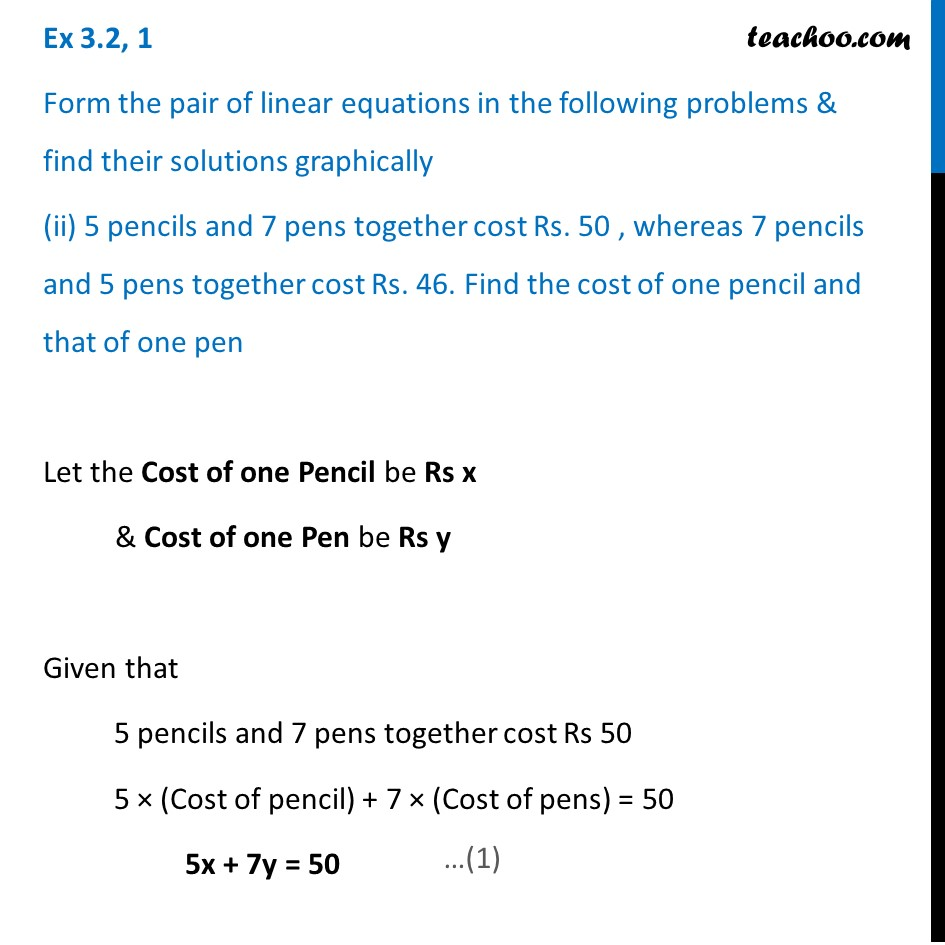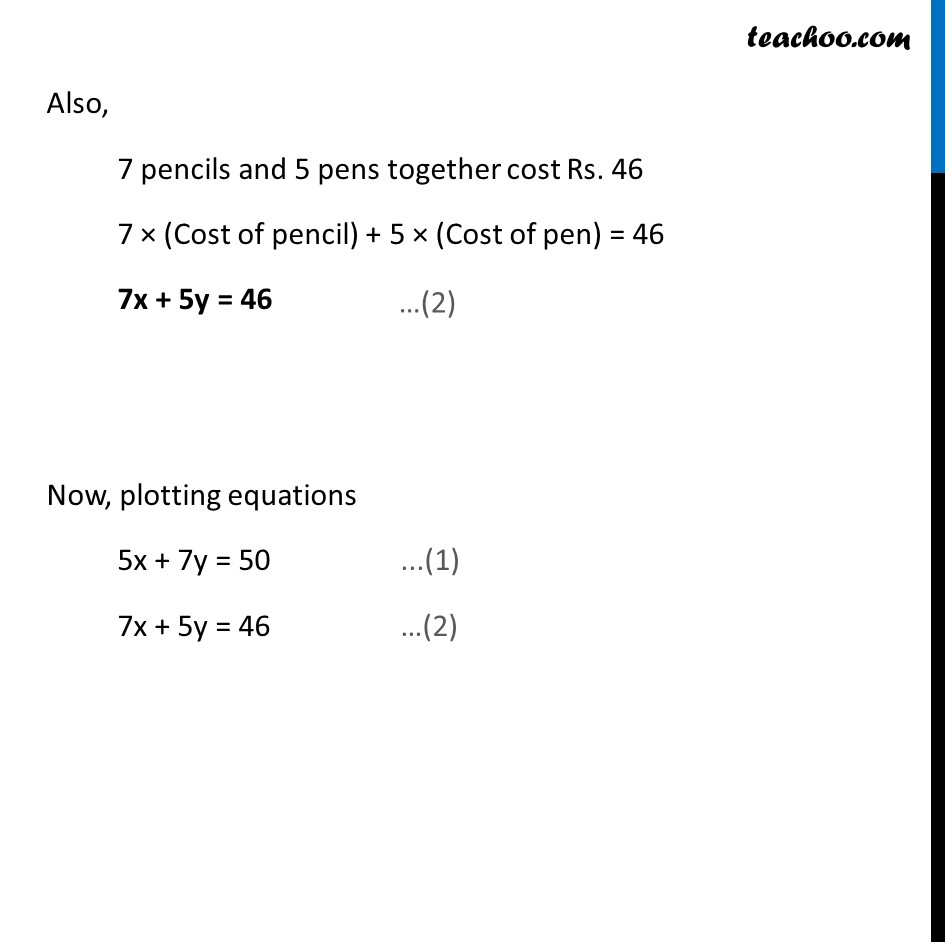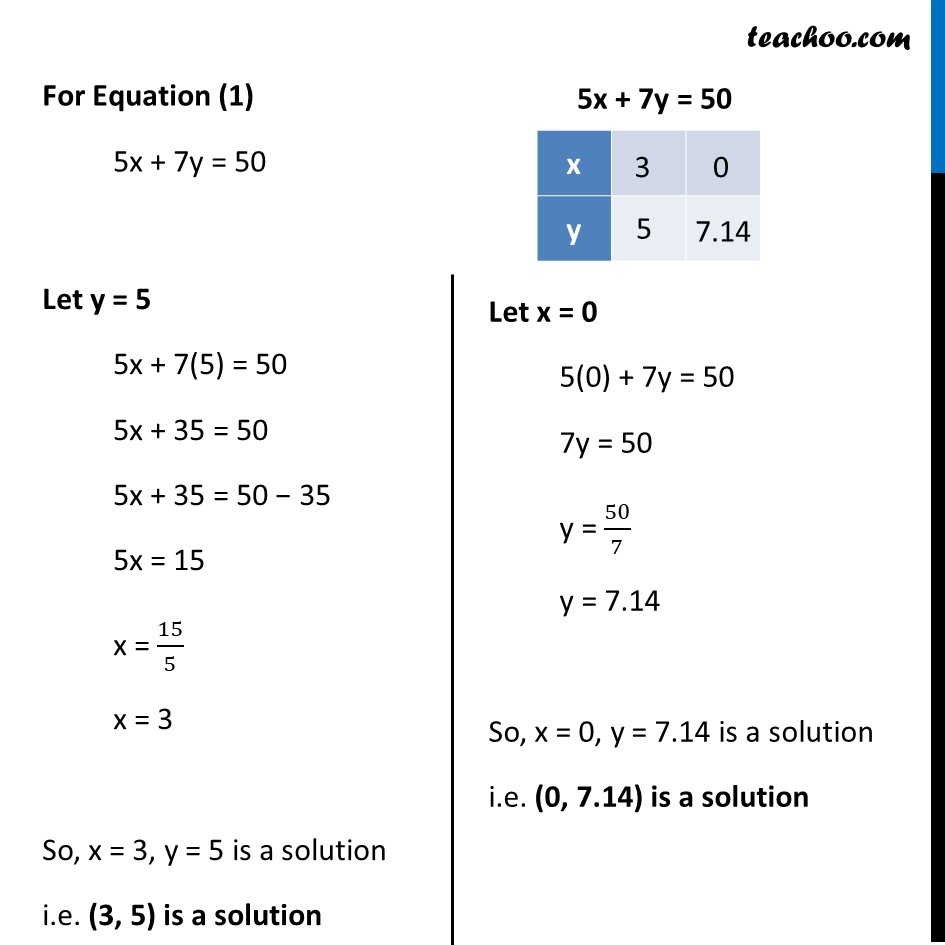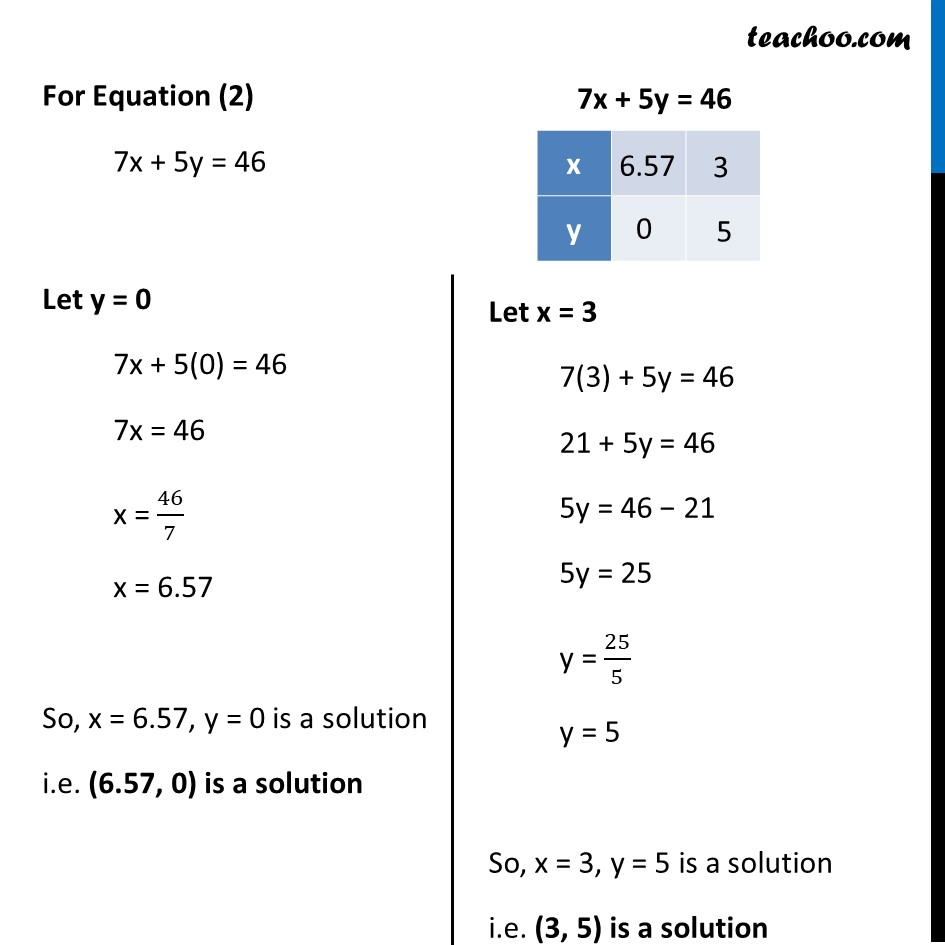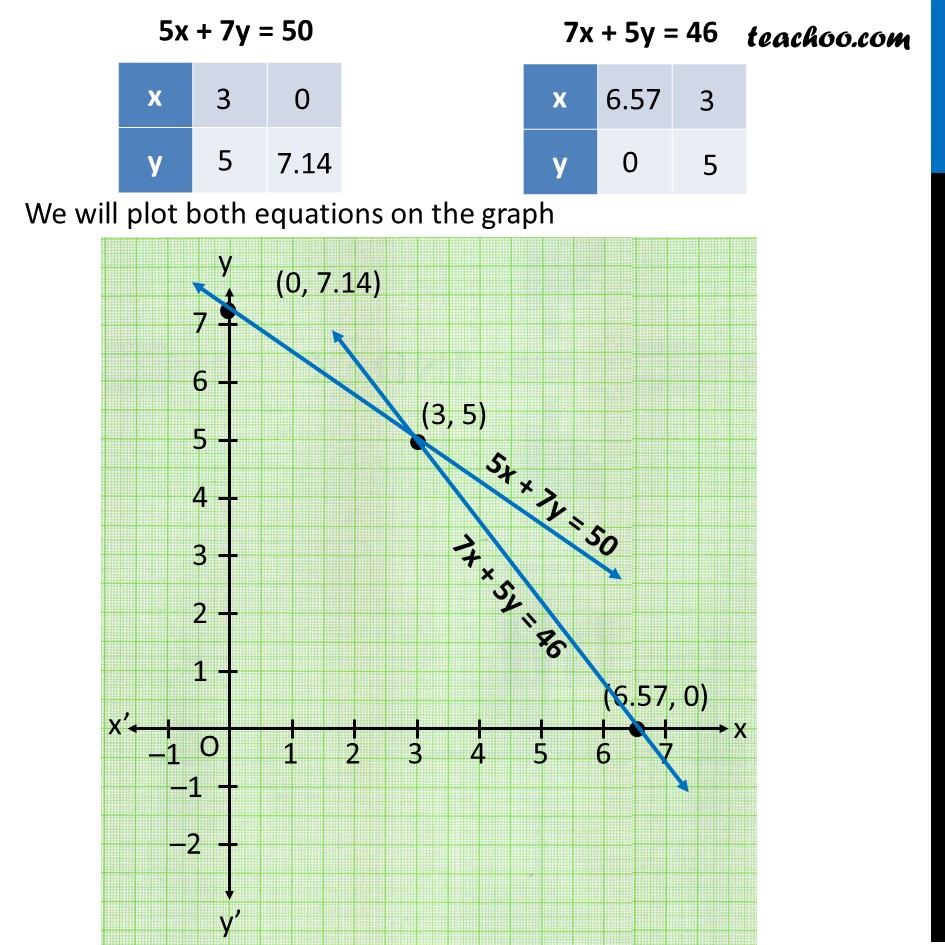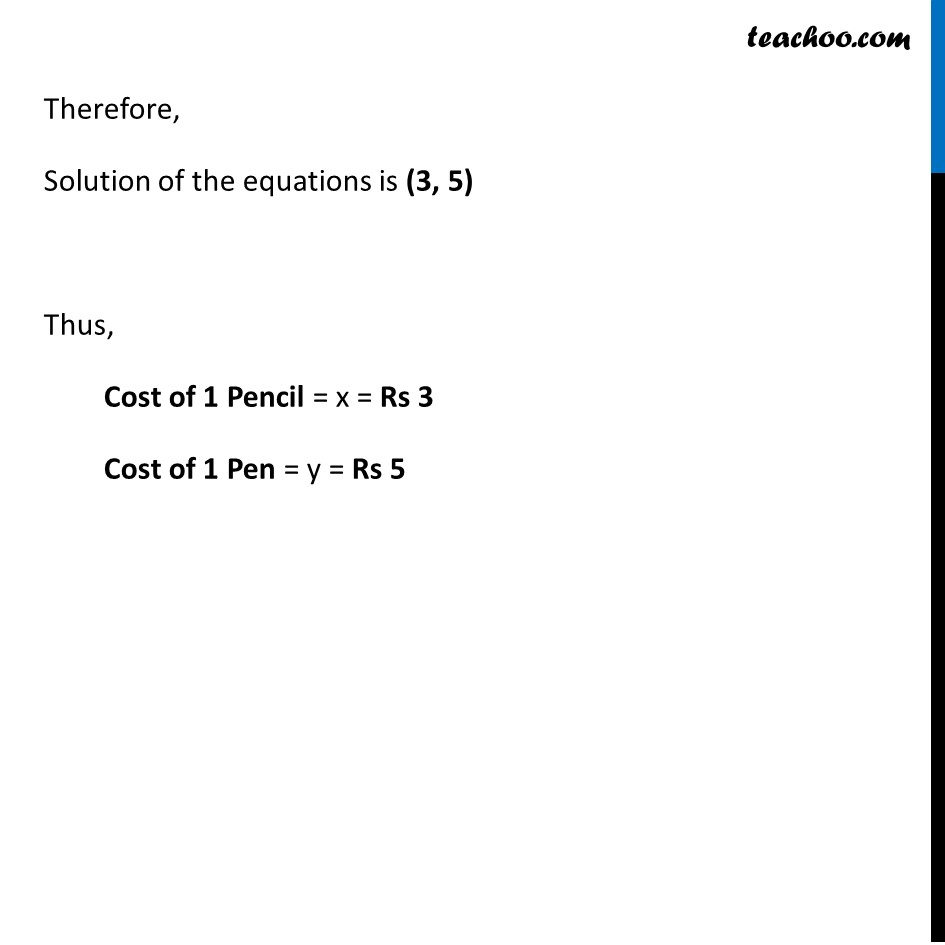Learn in your speed, with individual attention - Teachoo Maths 1-on-1 Class

### Transcript

Ex 3.1, 1 Form the pair of linear equations in the following problems & find their solutions graphically (ii) 5 pencils and 7 pens together cost Rs. 50 , whereas 7 pencils and 5 pens together cost Rs. 46. Find the cost of one pencil and that of one pen Let the Cost of one Pencil be Rs x & Cost of one Pen be Rs y Given that 5 pencils and 7 pens together cost Rs 50 5 × (Cost of pencil) + 7 × (Cost of pens) = 50 5x + 7y = 50 Also, 7 pencils and 5 pens together cost Rs. 46 7 × (Cost of pencil) + 5 × (Cost of pen) = 46 7x + 5y = 46 Now, plotting equations 5x + 7y = 50 ...(1) 7x + 5y = 46 …(2) For Equation (1) 5x + 7y = 50 Let y = 5 5x + 7(5) = 50 5x + 35 = 50 5x + 35 = 50 − 35 5x = 15 x = 15/5 x = 3 So, x = 3, y = 5 is a solution i.e. (3, 5) is a solution Let x = 0 5(0) + 7y = 50 7y = 50 y = 50/7 y = 7.14 So, x = 0, y = 7.14 is a solution i.e. (0, 7.14) is a solution For Equation (2) 7x + 5y = 46 Let y = 0 7x + 5(0) = 46 7x = 46 x = 46/7 x = 6.57 So, x = 6.57, y = 0 is a solution i.e. (6.57, 0) is a solution Let x = 3 7(3) + 5y = 46 21 + 5y = 46 5y = 46 − 21 5y = 25 y = 25/5 y = 5 So, x = 3, y = 5 is a solution i.e. (3, 5) is a solution We will plot both equations on the graph Therefore, Solution of the equations is (3, 5) Thus, Cost of 1 Pencil = x = Rs 3 Cost of 1 Pen = y = Rs 5